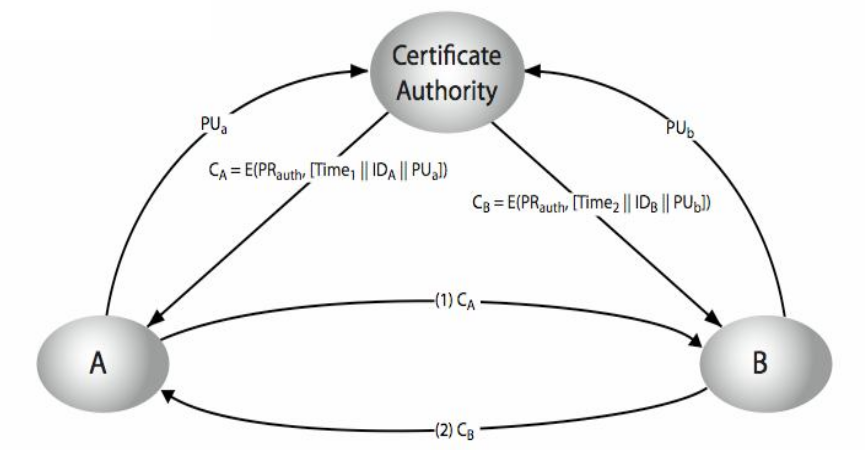NOTIFICATION: These examples are provided for educational purposes. The use of this code and/or information is under your own responsibility and risk. The information and/or code is given ‘as is’. I do not take responsibilities of how they are used. You are welcome to point out any mistakes in my posting and/or leave a comment.

RSA Algorithm

RSA is an algorithm for public-key cryptography. The signals R.S.A. come from the last name of Ron Rivest, Adi Shamir, and Leonard Adleman who where the first to describe this algorithm. This algorithm is famous for being the first suitable algorithm for signing as well as encryption.

RSA algorithm allow to choose which key should be use for encryption and decryption.

1. Public key for encryption, private key for decryption or,
2. Private key for encryption, public key for decryption.

Generate the Pair Key (Public and Private Key)

1. Choose two random prime numbers p and q.
p = 17
q = 11

For better security, you can use the Primality Test to obtain to obtain these two random prime number. They should be of similar bit-length.
2. Compute n = p*q in which n is the modulus used for both the private and public keys.
n = p * q = 17 * 11 = 187
3. Compute Euler Totient Function ø(n)
ø(n) = ø(187) = (p – 1) * (q – 1) = 16 * 10 = 160
4. Select a public key exponent e number where 1 < e < ø(n) and gcd(e, ø(n)) = 1
If we choose e = 7 then gcd(e, ø(n)) = gcd(7, 160) = 1
5. Determine the multiplicative inverse d: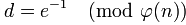1. d must be less than ø(n): d < 160
2. if d * e mod ø(n) = d * 7 mod ø(187) = d * 7 mod 160 = 1 then
3. let d = 23 in this way d * e = 23 * 7 = 161 = (160 + 1)
d * 7 mod 160 = 23 * 7 mod 160 = 1
6. The public key will be:
PU = {e, n} = {7, 187}
7. The private key will be:
PR = {d, n} = {23, 187}

Encryption

1. Sender must obtain the public key PU = {e, n} to the recipient, where PU is the public key, n for modulus, and e for public exponent (also known as public encryption).
PU = {e, n} = {7, 187}
2. The message M (also known as the plaintext) must be turn into an integer m by using a padding scheme (an reversible protocol) in which 0 < m < n.
Lets assume the message is m = 88 where 0 < m < n so 0 < 88 < 187.
3. Then the sender must compute the ciphertext.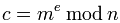Where c is the ciphertext, m is the integer message , e is the public exponent, and n i for modulus.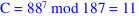Decryption

1. The recipient must use the private key to decrypt the ciphertext PR = {d, n} where PR is the private key, d is the private key exponent, n for modulus.
PR = {d, n} = {23, 187}
2. Compute the message.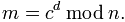Where m is the integer message, c is the ciphertext, n for modulus.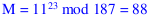3. Then turn back the original message M by using  integer message m with the reverse padding scheme.

Encryption / Decryption ExampleAlgorithm Requirements

1.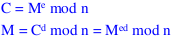2. There should be able to find values for e, d, and n so for all values of m where 0 < M < n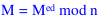3.andshould be easy to calculate for all valus of m where 0 < m < n.
4. It should be very hard for an attacker to determine d given e and n

Possible Attacks to RSA

1. Brute Attack
2. Mathematical attacks
1. Determine d directly
2. Determine the Euler Totient Function ø(n) without using the prime numbers p and q
3. Factorising n into the correct prime factors p and q

Key Distribution

One of the important aspects is how to distribute the keys between the sender and the receiver. For example, one way is to use the public-key encryption to distribute the keys.

For doing that there are three different methods of distributions that can be used:

1. Public announcement,
2. Public-key authority, and
3. Public-key certificates

Public Annoucement

One way to distribute the public keys is having the sender to distribute the public key to the recipient; however, this have the disadvantage that an attacker could create a key claiming to be the sender. This disadvantage is known as forgery.

A solution is to create a public-key autority.

Public Key Authority

A public key authority is a central authority that maintain a dynamic directory of public keys for all the users. Example: {name, public-key}

1. In a secure way (in person), each user register a public key in this directory authority.
2. It is required that the user known the public key for the directory.
3. Only the authority known the corresponding private key
4. Users interact with the directory in order to obtain the public key securely

Steps:

1. User A send  a timestamped message to the public key authority.
This message contain a request for the public key of user B.
2. The public-key authority responds to user A returning an encrypted message using it’s private key. This message contains:
1. The original request so it can be use to match with the request
2. The original timestamp so it can be determined if the message is not from the public-key authority.
3. The public key of user B.
3. User A store the public-key of user B and use this public-key to encrypt a message that will contain the identity of user A plus a “nonce N1”. This message will be deliver to user B.
4. User B send  a timestamped message to the public key authority.
This message contain a request for the public key of user A.
5. The public-key authority responds to user B returning an encrypted message using it’s private key. This message contains:
1. The original request so it can be use to match with the request
2. The original timestamp so it can be determined if the message is not from the public-key authority.
3. The public key of user A.
6. User B encrypt a message using the public-key of user A and send this encrypted message to user A.
This encrypted message have:

1. User A’s nonce
2. A nonce genereated by User B
7. User A encrypt a message using the public-key of User B and send this encrypted message to user B.
This encrypted message holds:

1. the  nonce N2 of user A

(This will ensure user B that the encrypted message is coming from user A).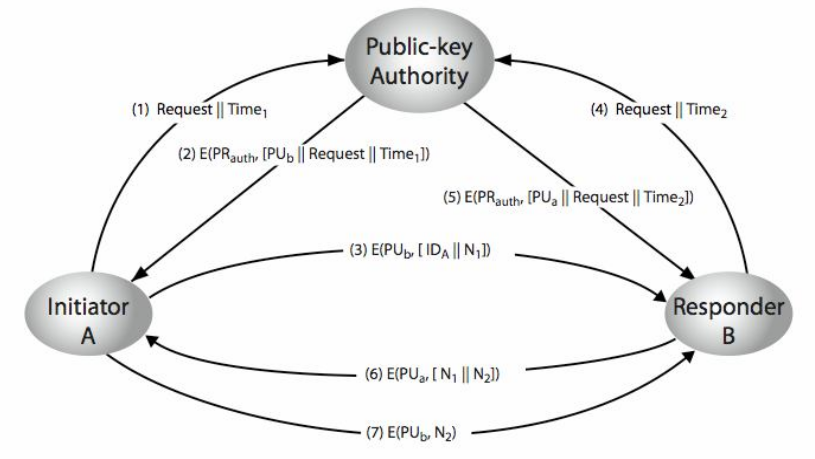Disavantages:

Since the users must appeal to the public-key authority in order to obtain the other users’ public key it can produce a bottleneck.

Public Key Certificates

Another way to exchange keys without the need of a public-key authority is the public-key certificates. The general idea would be:

1. A certificate is a data block that contains a public key plus an identifier of the key’s owner. This data block would be signed by a trusted third party which would be the certificate authority.
2. A user would generate a pair key and send the public key to this certify authority in a secure way and obtain a certificate issued by the certify authority (the trusted third party).
3. This user then would publish this certificate so another user can verify that the certificate was created by the trusted third party.

Please notice that the certificate authority (the trusted third party) is the only one that can create and update certificates.

Steps:

1. User A supply a public key PUa with a request for a certificate to the certificate authority. This request must be done in a secure ways such as in person for example.
2. The certificate authority would provide user A with this from: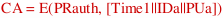where E is the encryption algorithm, PRauth is the authority’s private key and Time1 is a timestamp, and IDa is the user A identification.
3. User A then can pass the certificate CA any user (in this case user B).
4. User B get the certificate from user A and verify if the certificate correspond to the certify authority by decrypting the message using the authority’s public key: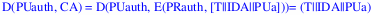In this way it can verify that the certificate is not counterfeit.